# 2. Array-Based Lists

In this chapter, we will study implementations of theandinterfaces where the underlying data is stored in an array, called the backing array. The following table summarizes the running times of operations for the data structures presented in this chapter:/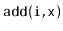/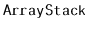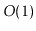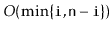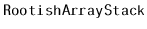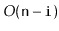Data structures that work by storing data in a single array have many advantages and limitations in common:
• Arrays offer constant time access to any value in the array. This is what allows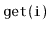andto run in constant time.

• Arrays are not very dynamic. Adding or removing an element near the middle of a list means that a large number of elements in the array need to be shifted to make room for the newly added element or to fill in the gap created by the deleted element. This is why the operationsandhave running times that depend onand.

• Arrays cannot expand or shrink. When the number of elements in the data structure exceeds the size of the backing array, a new array needs to be allocated and the data from the old array needs to be copied into the new array. This is an expensive operation.
The third point is important. The running times cited in the table above do not include the cost associated with growing and shrinking the backing array. We will see that, if carefully managed, the cost of growing and shrinking the backing array does not add much to the cost of an average operation. More precisely, if we start with an empty data structure, and perform any sequence of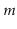oroperations, then the total cost of growing and shrinking the backing array, over the entire sequence ofoperations is. Although some individual operations are more expensive, the amortized cost, when amortized over alloperations, is onlyper operation.

In this chapter, and throughout this book, it will be convenient to have arrays that keep track of their size. The usual C++ arrays do not do this, so we have defined a class,, that keeps track of its length. The implementation of this class is straightforward. It is implemented as a standard C++ array,, and an integer,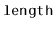:

```  T *a;
int length;
```
The size of anis specified at the time of creation:
```  array(int len) {
length = len;
a = new T[length];
}
```
The elements of an array can be indexed:
```  T& operator[](int i) {
assert(i >= 0 && i < length);
return a[i];
}
```
Finally, when one array is assigned to another, this is just a pointer manipulation that takes constant time:
```  array<T>& operator=(array<T> &b) {
if (a != NULL) delete[] a;
a = b.a;
b.a = NULL;
length = b.length;
return *this;
}
```

Subsections
opendatastructures.org